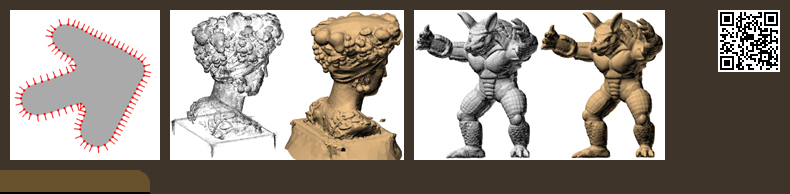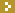###Abstract

We introduce a new variational formulation for the problem of reconstructing a watertight surface defined by an implicit equation, from a finite set of oriented points; a problem which has attracted a lot of attention for more than two decades. As in the Poisson Surface Reconstruction approach, discretizations of the continuous formulation reduce to the solution of sparse linear systems of equations. But rather than forcing the implicit function to approximate the indicator function of the volume bounded by the implicit surface, in our formulation the implicit function is forced to be a smooth approximation of the signed distance function to the surface. Since an indicator function is discontinuous, its gradient does not exist exactly where it needs to be compared with the normal vector data. The smooth signed distance has approximate unit slope in the neighborhood of the data points. As a result, the normal vector data can be incorporated directly into the energy function without implicit function smoothing. In addition, rather than first extending the oriented points to a vector field within the bounding volume, and then approximating the vector field by a gradient field in the least squares sense, here the vector field is constrained to be the gradient of the implicit function, and a single variational problem is solved directly in one step. The formulation allows for a number of different efficient discretizations, reduces to a finite least squares problem for all linearly parameterized families of functions, and does not require boundary conditions. The resulting algorithms are significantly simpler and easier to implement, and produce results of quality comparable with state-of-the-art algorithms. An efficient implementation based on a primal-graph octree-based hybrid finite element-finite difference discretization, and the Dual Marching Cubes isosurface extraction algorithm, is shown to produce high quality crack-free adaptive manifold polygon meshes.

###Acknowledgment of Support

The material presented in this web site describe work supported by the National Science Foundation under Grants No. CCF-0729126, IIS-0808718, and CCF-0915661.